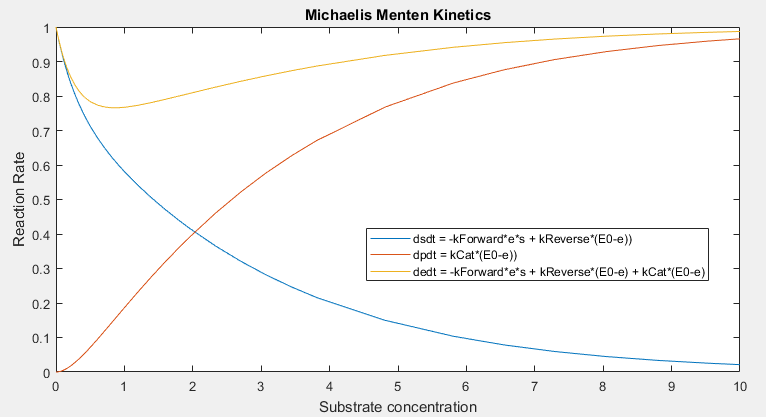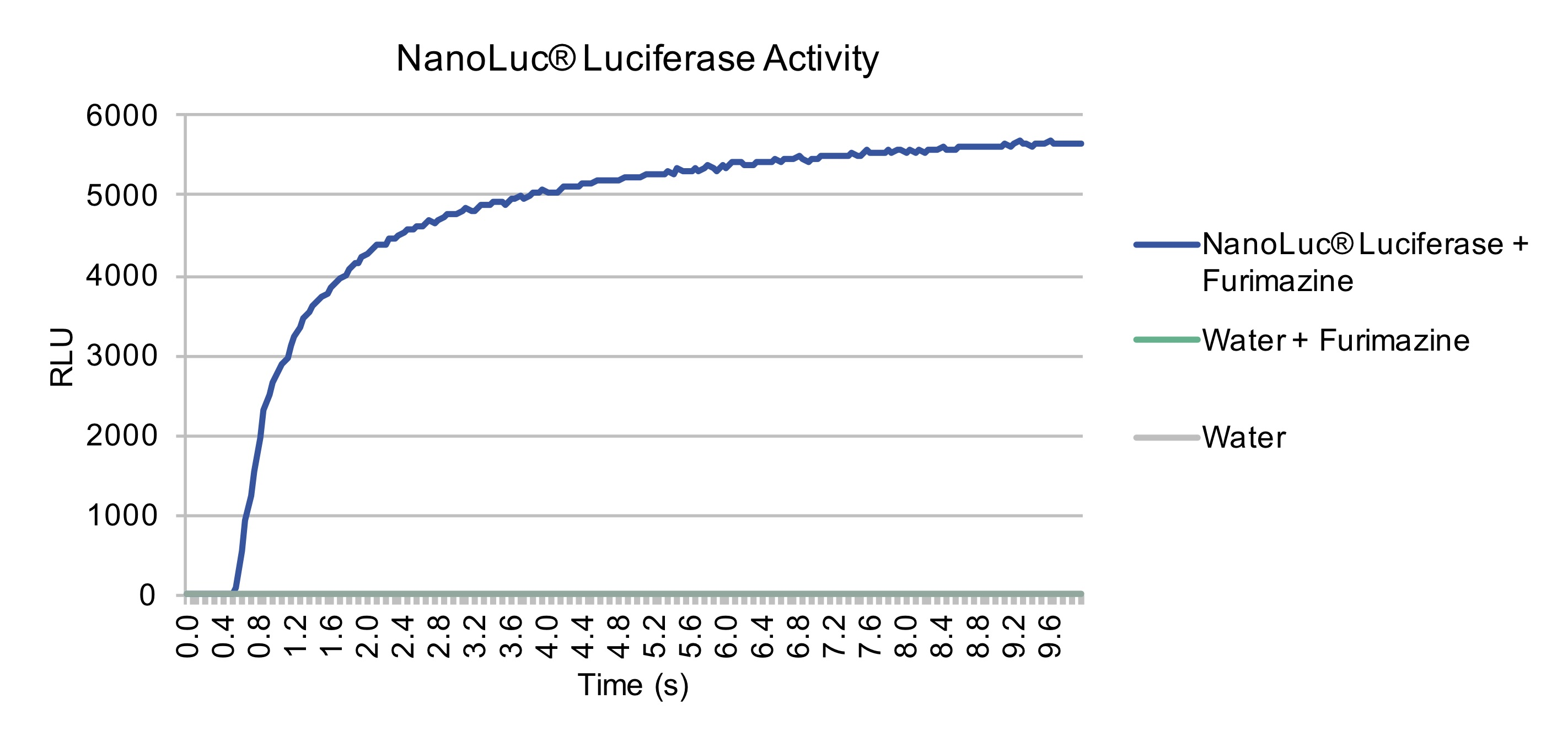## Michaelis-Menten Kinetics

### Introduction

Michaelis - Menten kinetics is a model used to examine enzyme kinetic. Luciferase's activity can be modeled by Michaelis-Menten kinetics as they perform the simple conversion of a substrate into a product and a photon. Our project relied on the light producing NanoLuc Luciferase as a signal in our devices. We were able to model this relationship with MATLAB. The governing equations for this model were compiled in the MATLAB, with the goal of creating a generic calculator which teams may use in the future. Known values for concentrations and reactions rates are used as inputs, and the file produces the various rates of change with respect to the concentrations.

### Governing Equations

The MATLAB code takes in the substrate concentration, product concentration, and enzyme concentration, represented as [S], [P] and [E] respectively. Additionally, known reaction rates for the forward, reverse, and catalytic directions are represented as kf, kr, and kcat respectively. The preceding values are put into a function which generates the rates of change of concentration with respect to time through the following first order ordinary differential equations:

d[E]/dt = -kf[E][S] + kr<[ES] + kcat[ES]
d[S]/dt = -kf[E][S] + kr<[ES]
d[P]/dt = kcat[ES]

The following substitution is used, where [E0] is the final substrate concentration

[E0]-[E]=[ES]

This yields the formulas used in the function, which are as follows

d[E]/dt = -kf[E][S] + kr<[E0-E] + kcat[E0-E]
d[S]/dt = -kf[E][S] + kr<[E0-E]
d[P]/dt = kcat[E0-E]

### Application

We sought to apply the calculator generated here to model the Kinetics of NanoLuc Luciferase, our choice biosensor reporter. As can be seen from the graph generated by this calculator, and by the actual results obtained. NanoLuc Luciferase activity can be very accurately modeled with this tool, since its activity is substrate dependent, eventually reaching its maximum reaction rate when [S] >> [E].Michaelis-Menten Model of Enzyme Kinetics, demonstrating reaction rate is dependent on the concentration of a substrate S.NanoLuc Luciferase activity is dependent on substrate concentration. At T=0, an excess of substrate was added. Over the next 10 seconds, the activity of NanoLuc Luciferase increases to a plateau, by converting the substrate Furimazine, into Furimamide, light and CO2

### Use

To use the calculator, simply open the MATLAB file and enter the reaction rates and concentration in the noted areas. After running the file, the reaction rates will appear in the MATLAB command window.

### Matlab Code

%********READ ME********

%The following is a simple Michaelis–Menten rate of change calculator
%The commented out portion of the code is just the governing equations
%Fill in the parameters of dXdT in terms of s,p, and e
%Fill in the reaction rates (all set to 1 by default)
%The output will be the various rates

%Governing Equations

%E + S <> ES > E + P
%s=[S];
%p=[P];
%e=[E];
%c=[ES];

%ds/dt = -kForward*e*s + kReverse*c
%dp/dt = kCat*c
%de/dt = -kForward*e*s + kReverse*c + kCat*c
%dc/dt = kForward*e*s - kReverse*c - kCat*c

%de/dt + dc/dt = 0
%d(e+c)/dt = 0

%E0 = e + c

%ds/dt = -kForward*e*s + kReverse*(E0-e)
%dp/dt = kCat*(E0-e)
%de/dt = -kForward*e*s + kReverse*(E0-e) + kCat*(E0-e)

s = 1; %Enter substrate concentration
p = 1; %Enter product concentration
e = 1; %Enter enzyme concentration

dXdT([s p e])

function [f] = dXdT(x)

kForward = 1; %Enter forward reaction rate
kReverse = 1; %Enter reverse reaction rate
kCat = 1;     %Enter catalytic reaction rate
E0 = 1;       %Enter initial concentration

s=x(1);
p=x(2);
e=x(3);

dsdt = -kForward*e*s + kReverse*(E0-e);
dpdt = kCat*(E0-e);
dedt = -kForward*e*s + kReverse*(E0-e) + kCat*(E0-e);

f = [dsdt; dpdt; dedt];
end

%***Acknowledgments***

%Daniel Beard - Enzyme Kinetics with MATLAB 2